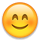Mileage Points

Weekly Mission = 1050*4 = 4200
Daily Mission = 360*30 = 10800
MISSIONS = 4200 + 10800 = 15000

ATTENDANCE CHECK:
1800+450+450+5600 = 8300
30+50+70+120 = 450 + 180*24 = 4770
TOTAL = 13070

FEVER BAR

Fever Section Change 10 MP x 6 times per day 60*30 = 1800
Fever Bar Bonus for Fri/Sat/Sun 180*14 = 2520

TOTAL = 2520+1800 = 4320

....

15000+13070+4320 = 32390 !Also Fever Bar is supposed to give these bonuses only at Fri/Sat/Sun but it seems like it works on the other days ..
Bugged version gives 180*30 = 5400 + 1800 for Fever section which is total of 7200 in 30 days and 7440 when month has 31 day
Also 360 more for Daily mission which is total

7800 more with in months with 31 day and with bugged Fever system :P

Hope it helps and saves someone's time hehe